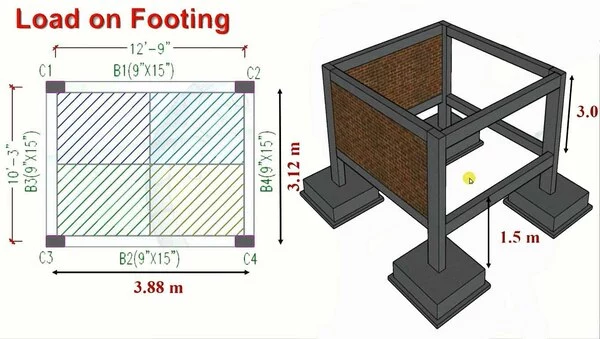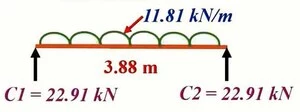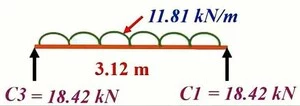# How to Calculate Load on Footing for Structural Support

In this blog post, we will explain how to calculate load on building footing and the different types of loads acting on it. We will also cover the formula and calculation used in the process, as well as the factors that can affect the load on a building footing or foundation load calculation. The post will also provide insight on why it’s important to consult a professional structural engineer for the proper calculation of loads on building footing and the foundation design.

Footing is one of the major structural elements that transfer the load safely to the soil. The slab load will transfer to the beam load, which will transfer to the column. The column will transfer the load to the footing, which has to safely transfer the load to the soil.

## What is Footing?

It is the lowermost part of the foundation that has been constructed below Ground level in solid surface.

## Purpose of footing?

Transfer the live load and dead loads of the structure over a large enough area so that neither the soil nor the building moves. Resist settlement and lateral load.

## Load Calculation Procedure for Footing:

Before we begin the calculation, let’s take a look at the loads that will be applied to the footing.### Calculate Load acting on Footing | Calculation Example

5. Self Weight of Footing

### Given Structural Dimensions:

• Column Size = 9″ x 15″
• Beam Size = 9″ x 15″
• Slab Thickness = 5″
• Size of Footing = 4′ x 4′ x 12

### 1- Calculate Load from Slab:

Slab Thickness = 5″ = 0.125m

Footing Calculation Formula: Self Weight of Slab = Volume of Slab x Unit Weight of Concrete

Self Weight of Slab = (1 x 1 x 0.125) x 25

Self Weight of Slab = 3.125 KN/m2

Floor Finish Load = 1.5 KN/m2

Total Load on Slab = 3.125 + 1.5 + 2.0 = 6.625 KN/m2

Influence Area (C1) = L/2 x W/2

Influence Area (C1) = 3.88/2 x 3.12/2 = 1.94 x 1.56

Influence Area (C1) = 3.03 m2

Slab Load on Column = Total Load on Slab x Influence Area (C1)

Slab Load on Column = 6.625 x 3.03

Slab Load on Column = 20.07 KN

### 2- Calculate Load from Beam:

Beam Size = 9″ x 15″ = 0.225 m x 0.375 m

Footing Calculation Formula: Self Weight of Beam = W = Unit Volume of Beam x Unit Weight of Concrete

Self Weight of Beam = W = (1 x 0.225 x 0.375) x 25

Self Weight of Beam = W = 2.11 KN/m

B1 Beam Load on Column C1/C2 = WL/2

B1 Beam Load on Column C1/C2 = (2.11 x 3.88)/2

B1 Beam Load on Column C1/C2 = 4.09 KN

B3 Beam Load on Column C1/C3 = WL/2

B3 Beam Load on Column C1/C3 = (2.11 x 3.12)/2

B3 Beam Load on Column C1/C3 = 3.29 KN

Total Load on C1 from Beams = 4.09 + 3.29

Total Beam Load on C1 from Beams = 7.38 KN

### 3- Calculate Load from Plinth Beam & Wall:

Plinth Beam Size = 9″ x 15″ = 0.225 m x 0.375 m

As we know that the size of beam is same as Plinth Beam so the Total Load on C1 from Plinth Beam are also same to the Total Load on C1 from Beams so,

Total Load on C1 from Plinth Beams = 7.38 KN

Height of Wall = Overall Height – Beam Depth

Height of Wall = 3 – 0.375 = 2.625 m

Footing Calculation Formula: Self Weight of Wall on Plinth Beam = W = Volume of Wall x Density of Bricks

Self Weight of Wall on Plinth Beam = W = (1 x 0.225 x 2.625) x 20

Self Weight of Wall on Plinth Beam = W = 11.81 KN/mB1 Wall Load on Column C1/C2 = WL/2

B1 Wall Load on Column C1/C2 = (11.81 x 3.88)/2

B1 Wall Load on Column C1/C2 = 22.91 KNB3 Wall Load on Column C1/C3 = WL/2

B3 Wall Load on Column C1/C3 = (11.81 x 3.12)/2

B3 Wall Load on Column C1/C3 = 18.42 KN

Total Load on C1 from Walls = 22.91 + 18.42 = 41.33 KN

### 4- Calculate Load from Column:

Column Size = 9″ x 15″ = 0.225 m x 0.375 m

Height of Column = (Height Above Ground + Hight Below Ground) = 3 + 1.5 = 4.5 m

Footing Calculation Formula: Self Weight of Column = W = Volume of Column x Density of Concrete

Self Weight of Column = W = (0.225 x 0.375 x 4.5) x 25

Self Weight of Column = W = 9.49 KN

### 5- Calculate Self Weight of Footing:

Size of Footing = 4′ x 4′ x 12″ = 1.22 m x 1.22 m x 0.30 m

Footing Calculation Formula: Self Weight of Footing = W = Volume of Footing x Density of Concrete

Self Weight of Footing = W = (1.22 x 1.22 x 0.30) x 25

Self Weight of Footing = W = 11.16 KN

Volume of Footing = 1.22 x 1.22 x 0.3 = 0.446 m3

As we know that the size of footing excavation = 4′-4″ x 4′-4″ x 1.5= 1.32 m x 1.32 m x 1.5 m

Volume of Footing Excavation = 1.32 x 1.32 x 1.5 = 2.61 m3

Volume of Filling = Volume of Footing Excavation – Volume of Footing

Volume of Filling = 2.61 – 0.446 = 2.164 m3

As we know that Unit Weight of Earth = 18 KN/m3

Backfilling Load = Volume of Filling x Unit Weight of Earth

Backfilling Load = 2.164 x 18 = 38.95 KN

1. Load from Slab = 20.07
2. Load from Beam = 7.38
3. Load from Plinth Beam & Wall = 7.38 + 41.33 = 48.71 KN
4. Load from Column = 9.49 KN
5. Self Weight of Footing = 11.16 KN
6. Backfilling Load = 38.95 KN
so, Total Load acting on Footing = 135.76 KN

## Conclusion:

In conclusion, determining the load on a building’s footings is an important phase in the building process. The building’s footings can withstand the weight of the structure if the calculations are done correctly, averting expensive and dangerous structural problems. The load on a building’s footings can be determined in a number of ways, including manually, via advanced structural design software, and by consulting an expert. It’s crucial to pick the best approach that works for your particular building project.

## What is a load on a building footing?

A load on a building footing is a force applied to the building's foundation, encompassing the weight of the structure and its contents as well as any outside influences like wind, snow, and seismic activity.

## How are loads on building footings calculated?

In order to determine the loads on building footings, a mix of engineering concepts, building codes, and calculations based on the unique design and location of the building are usually used. For the correct calculation, it's critical to seek the advice of a qualified structural engineer.

## Is it necessary to consult a professional for calculating the load on building footings?

Yes, In order to accurately calculate the loads on building footings and construct a foundation that can support them, it is advised to seek the advice of a professional structural engineer. They have the knowledge, training, and experience needed.

## How are loads distributed in structural system?

The loads are transferred from walls via slabs and beam functions to vertical wind beams and then further to the gable roof areas. Through the pores of the roof area the loads are transferred to wind swaying's and the tension carries on to the foundation of the façade.

## How do you calculate footing size for a load?

For measuring footing dimensions one can use simple calculations: structural loading (lbs) / soil - capacity (ppf) = footing area needed (ft. x m).

1.Noor Ahmad Mahmoodi

Send me

• Thanks for the comments please visit more articles by clicking on “All Posts” tab of the Menu Bar

2.Whennie Pepito

It’s good and very useful for the civil engineers

• Thanks for the comments please visit more articles by clicking on “All Posts” tab of the Menu Bar

3.ABRAR

SIR,PLS AN ARTICAL TO CALACULTE THE STONE MASONRY WALL DESIGNE WITH ALL RESPECT